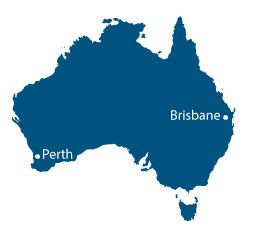# Year 5

## Measurement and Geometry

### Lengths and areas

In Australia we use the metric system of measurement. The units commonly used to measure length are the millimetre, centimetre, metre and kilometre.

The metre is the basis of the other units of length measurement. The prefix before the word metre tells us about the size of the unit:• centi tells us that 1 centimetre is $$\dfrac{1}{100}$$ of a metre
• milli tells us that 1 millimetre is $$\dfrac{1}{1000}$$ of a metre
• kilo tells us that 1 kilometre is 1000 times a metre.

When choosing units it is important to choose the appropriate unit for the task. The length of a block of land would be measured in metres, the width of a piece of paper might be measured in millimetres or centimetres, and the distance from Brisbane to Perth would be measured in kilometres. Builders always make measurements in millimetres, to ensure that their measurements are accurate and to avoid making mistakes because of muddled units.

We can calculate the perimeter of an object by measuring around it. The units of measurement for perimeter are the same as those used for length.

Measuring area allows us to describe and compare the amount of surface an object occupies. For example, we can compare the area of two blocks of land to decide which is larger.